# Team:Grenoble-EMSE-LSU/Project/Modelling/Density

Grenoble-EMSE-LSU, iGEM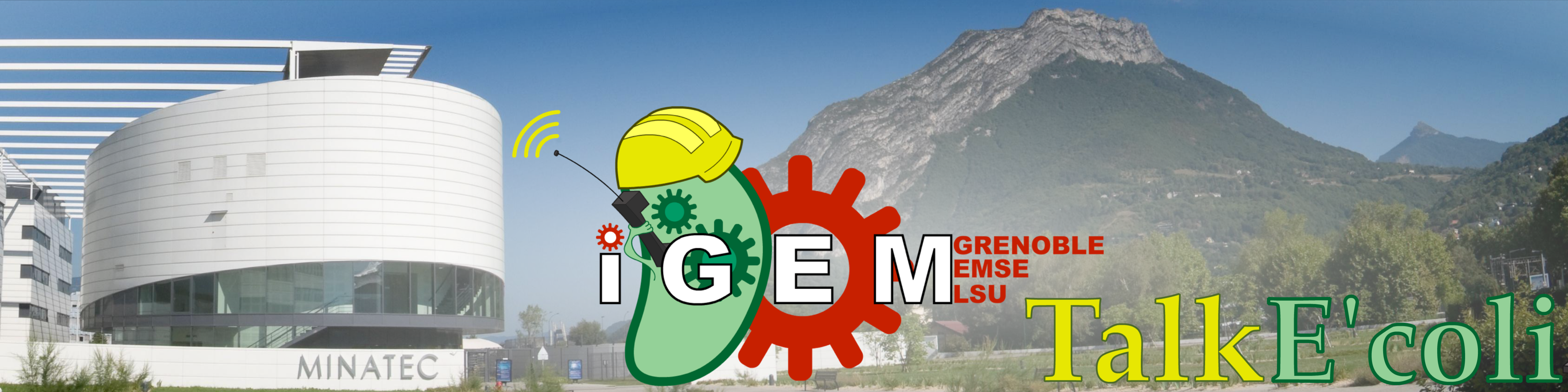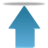Grenoble-EMSE-LSU, iGEM

• # Density Control

The aim of our project is to control the density of a living bacterial population with light-transmitted signals:

$\bullet$From the cells to computer: fluorescence and backscattered light as measurable signals from the cell suspension.

$\bullet$From the computer to the cells: white illumination to kill cells expressing KillerRed proteins. Its intensity needs to be adjusted.

The density of living cells cannot be measured instantaneously: it is determined by plating the cells on LB-agar plates and counting the colonies.

In order to validate our system, we first tried to have a constant population of living bacteria. This situation is characterized by an $OD_{600}$ growing up in a linear way (see below). Our model was necessary to find the best time profile of the illumination $I(t)$ to reach as quickly as possible a stable level of the amount of living bacteria

• ## Stabilization of the living cell density by light

In ourinitial model we showed that it was theoretically possible to stabilize the amount of living bacteria with a constant light intensity. With the complete model, this still holds true, as shown by the following simulations. In addition, numerical simulation shows that the light intensity is a very sensitive parameter. Below are displayed three simulated 16-hours long kinetics, for a cell suspension illuminated at different percentage of the maximal illumination:

1. $25\%$ for the green one.

2. $30\%$ for the cyan one.

3. $32.7\%$ for the blue one.

4. $35\%$ for the magenta one.

5. $100\%$ for the red one.

These simulations were conducted with the following values of the parameters:

$r=8,3.10^{-3} min^{-1}$, or $R=83 min$ (time of division)

$a=130 RFU.OD^{-1}.min^{-1}$

$b=0,9.10^{-2}RFU.UL^{-1}.min^{-1}$

$k=0,9.10^{-7}OD.RFU^{-1}.UL^{-1}.min^{-1}$

$l=0.087$

$m=6,3.10^{-3} min^{-1}$, or $M=110 min$ (half-time of maturation)

And the initial contitions were:

$OD_{600}=0.015$

$fluorescence=0RFU$

In this first figure, the density of living cells $C(t)$ is displayed in $OD_{600}$ units. When the light intensity is too strong, all bacteria die, when it is too weak, they grow exponentially. A particular value of the light intensity allows to get a constant density of living cells. Note that this light intensity $I^*$ should be precisely regulated, since a 2.5% increase or decrease around this particular light intensity will result in a 30% decrease or increase of the living cell density after 10 hours.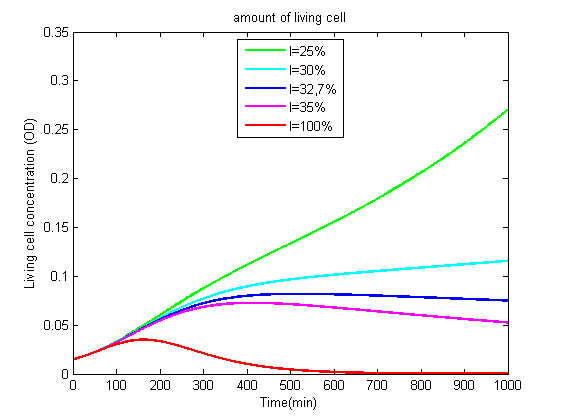The evolution of $OD_{600}$ is due to both living and dead bacteria. When the light intensity is too strong, the OD600 tends to a constant, when the light intensity is too weak, it growths exponentially. At the light intensity $I^*$, $OD_{600}$ increases linearly.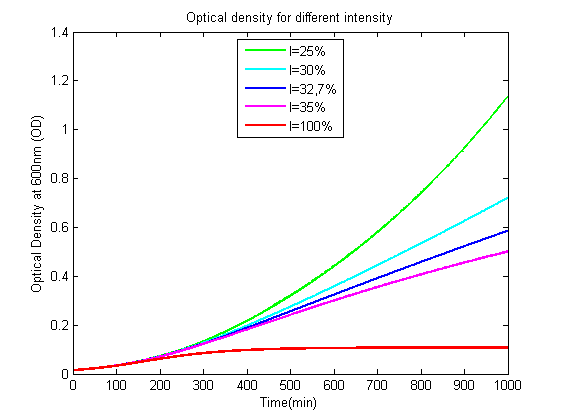The evolution of KillerRed fluorescence is also very sensitive to the light intensity. It decreases when the illumination is too strong, increases rapidly when it is too weak, and increases more slowly at the stabilizing light intensity $I^*$.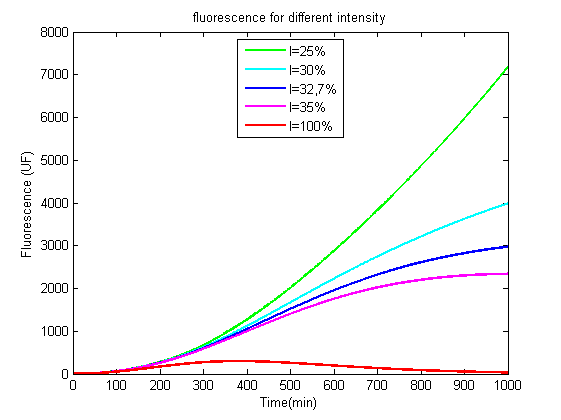Since even small deviations from the stabilizing light intensity $I^*$ results in large variations of cell density, an iterative process was designed to determine it with high precision.

• ## Model Predictive Control

Since our system cannot be stabilized with a simple closed-loop control, we used a more advanced control method: Model Predictive Control. This algorithm is used to control dynamical systems. It requires a mathematical model of the behavior of the system, and the history of the past control actions. The aim is to drive our system to a setpoint by predicting the evolution of the system, and adapting the control parameter (the light intensity here) to these predictions. To do so, the control will use different kinds of variables:

$\bullet$ Some variables defines the setpoint, here, we want to drive our living bacteria concentration to a determinate value, called $C_{target}$.

$\bullet$ Some variables are measurable during the experiment. Here, the fluorescence and the optical density. They are the only observable quantities enabling us to see whether the system behaves according to plans or not.

$\bullet$ Other variables cannot be measured and have to be estimated from the model. In our case, the concentration of living bacteria cannot be measured in real time, so it will be estimated from the measurable quantities. The model thus needs to be precise.

$\bullet$ And some variables are the ones used to act on the system. In our case, this is the light intensity which will affect the system by decreasing the amount of living bacteria and the concentration of KillerRed.

Here are the outlines of the algorithm:

$1$. To start with, cells are grown in the dark before the onset of the experiment. We thus have full information on the system because the fluorescence $K(0)$ and the $OD_{600}$ of $C(0)$ correspond to living cells only.

$2$. An illumination $I_1(t)$ is calculated, which according to the model is supposed to drive $C(t)$ to its setpoint $C_{target}$. The total fluorescence $F_1(t)$ and the living cell $C_1(t)$ kinetics are also computed.

$3$. For a certain amount of time $\tau$, more than 10 minutes to see the effect of the illumination, light is applied to the cell suspension at intensity $I_1(t)$

$4$. At time $t=\tau$, the real fluorescence, $F(\tau)$, is measured and compared to the estimated one, $F_1(\tau)$.

$5$. Others hidden variables as $C(\tau)$ are estimated using to the difference between $F(\tau)$ and $F_1(\tau)$. If $F(\tau)< F_1(\tau)$, it means hat we overestimated cell growth, and thus $C_{real}(\tau)< C_1(\tau)$.

$6$. From the estimated and measured values of $C$, we recalculate the value of the illumination : $I_2(t)$, $F_2(t)$ and $C_2(t)$ are created and the algorithm loops to step $2$ .

This algorithm will not perfectly drive $C(t)$ to its setpoint $C_{target}$. Imperfections in the model will create deviations. But our proof of concept has shown that these are not too big compared to the value of $C_{target}$.

• ## Heuristic method to Control the density of living cells in a bacterial population

We previously observed that some of the parameters of our model ($b$, $k$ and $l$) were quite variable, from experiment to experiment. Since the quality of the predictions greatly depends on them, it is important to determine them accurately. We therefore designed a procedure to obtain them during each experiment.

$1$ Cells are first incubated in the dark during a first period of approximately 100 minutes without illumination to let the bacteria grow and produce KillerRed.

$2$ During a second period, the cell suspension was illuminated at maximal intensity ($I=1$). This makes it possible to precisely determine the capricious parameters ($b$, $k$ and $l$). The length of this period depends on the precision wanted for the parameters: the longer it lasts, the largest the effects of light are, and more efficiently is the fit improved.

$3$ Once the parameters are chosen, the model is used to determinate the light intensity predicted to stabilize our system.

$4$ Each time the measured values of fluorescence or absorbance are too far from the predicted ones, parameters are re-estimated, and so is the light intensity that is supposed to stabilize the system. This stabilization could be automatically performed by the model predictive control. We instead run our model to determine changes to apply to the light intensity to reach the desired cell density value

Another fact should be taken into consideration. We want to stabilize the living cell density in the minimum time, because otherwise the cell culture may become contaminated or run out of nutrients. We empirically observed that the two first steps of our procedure are essential to speed up the stabilization of the living bacteria population. If the light is set at the stabilizing value $I^*$ from the beginning, the living cell density stabilizes after 8 hours (see the predition of living cells). Exposing the bacteria to a large intensity, then reducing the light, makes the living cell population to stabilize more quickly.